# Kelvin functions

Thomson functions

The functions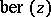and,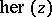and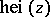,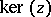and, defined by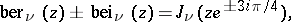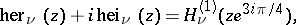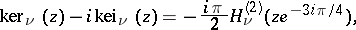where theare the Hankel functions and the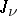are the Bessel functions. When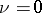the index is omitted. The Kelvin functions form a fundamental system of solutions of the equation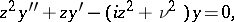which forturns into the Bessel equation.

The series representations are: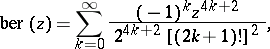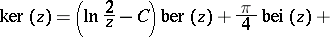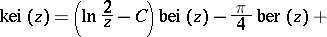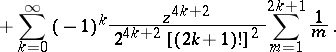The asymptotic representations are: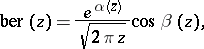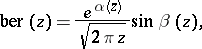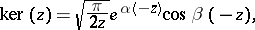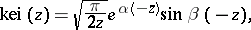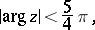where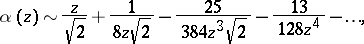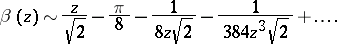These functions were introduced by W. Thomson (Lord Kelvin, ).